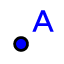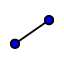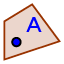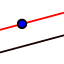# An exploration of ratios of parts of a triangle

Topic:
Ratios
1. Use the pointand segmenttools to create a scalene triangle with vertices A, B, and C.
2. Use the point on object toolto put point D anywhere on side AC.
3. Use the parallel line toolto construct a line parallel to side AB through D.
4. Use the intersect toolto create point E where your parallel line intersects side BC.
5. Use the show/hide object toolto hide line DE and segments AC and BC. (This tool will give the impression it's just turning these part gray, when you click on the move tool again, they'll actually disappear
6. Use the segment toolto create segments DE, AD, CD, BE, and CE and use the move tooland style bar to change the colors of these segments to easily distinguish which is which in the algebra window.
7. Note that the length of each segment is listed along with a letter to represent it in the algebra window. Use the appropriate combinations of these letters in the input bar (at the bottom of the algebra window) to calculate the ratios and . The results of these calculations will appear as numbers near the top of the algebra window.

Use the move toolto drag around all draggable points in the diagram, keeping an eye on the ratios you had GeoGebra calculate for you in Step 7. What do you notice?

Propose a theorem to summarize what you've observed in this construction.